June 27, 2016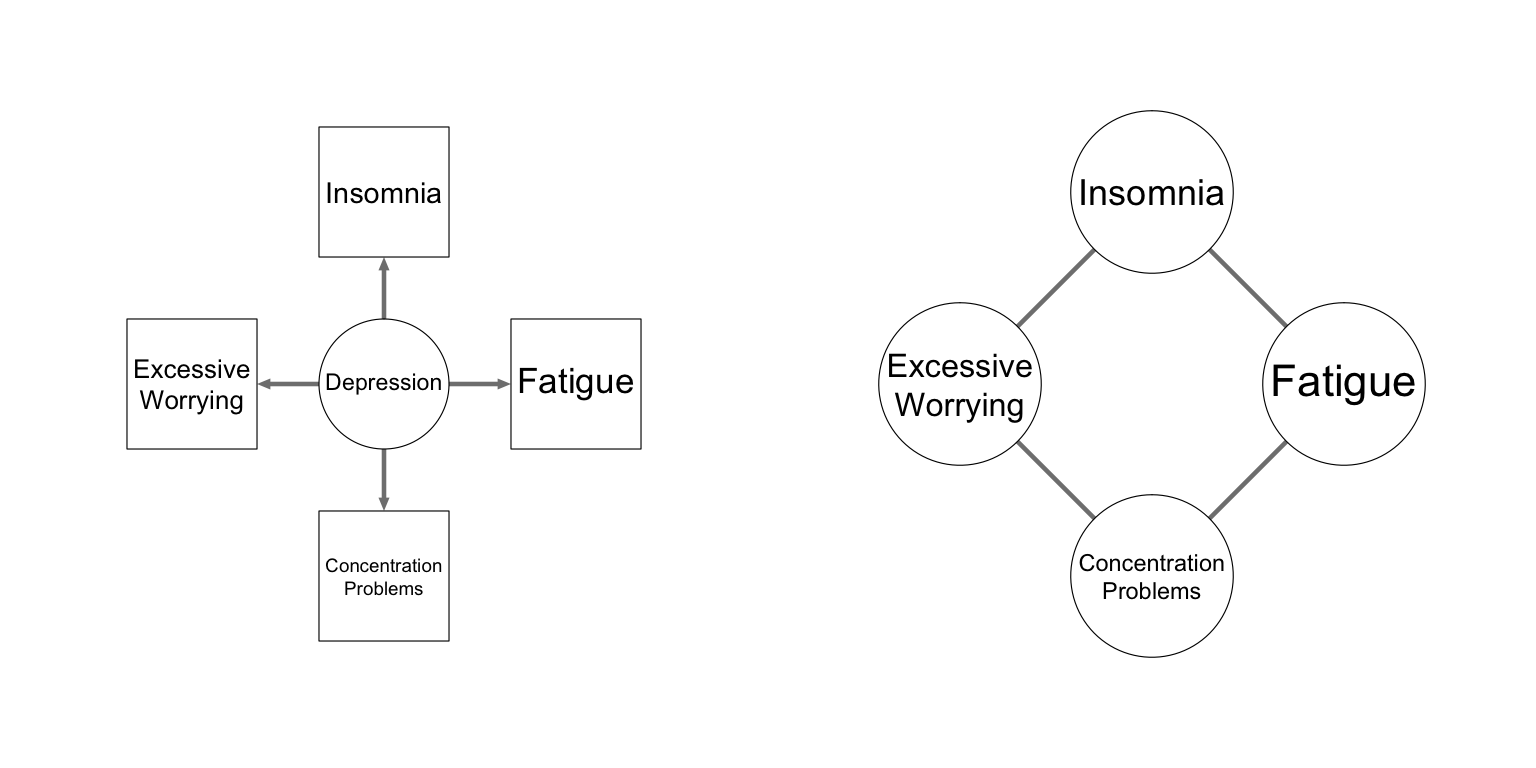## The Dynamics of Psychology

• Psychological constructs can be conceptualized as dynamical systems, featuring complex emergent behavior:
• Correlated responses
• Stable "traits"
• Phase transitions
• Individual Differences
• These systems can be portrayed as networks
• As network structures are unknown in psychology, they need to be estimated
• Network Psychometrics

## Goal

• Up to three network structures can be obtained in time-series data:
• Contemporaneous networks
• Temporal networks
• Between-subjects networks
• All three structures can be interpreted in several ways:
• Highlighting potential causal pathways
• Showing predictive effects and mediation
• Under very strict assumptions: a causal model

## Outline

• When cases are independent
• The Gaussian Graphical model
• Interpreting network structures
• When cases are not independent: $$N = 1$$
• The VAR model
• Temporal and contemporaneous networks and causation
• When cases are not independent: $$N > 1$$
• The multi-level VAR model
• Between-subjects networks and causation
• Conclusion

## Experience Sampling Method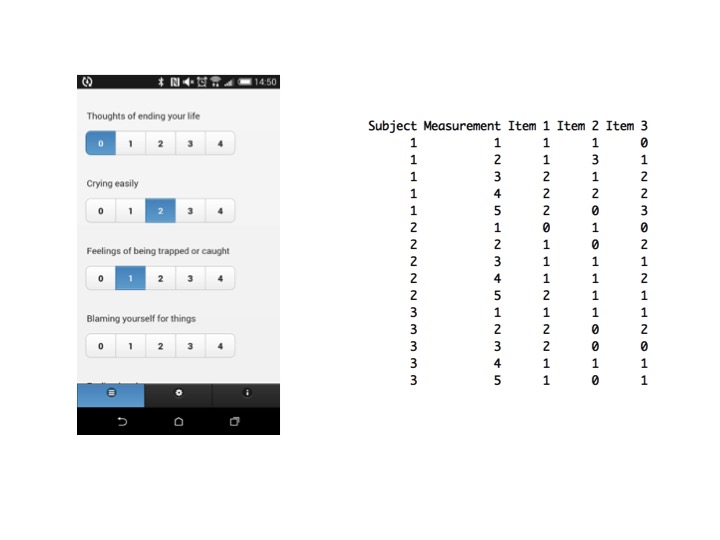• Rows are termed the cases

## Cross-sectional Data

• Every person measured only once
• Cases can reasonably be assumed to be independent
• Given IQ has a mean of 100 and SD of 15, does knowing that Peter has an IQ of 90 help us predict better that Sarah had an IQ of 110?
• Because of this assumption, likelihood reduces to a product
• $$\pmb{Y} \sim N\left(\pmb{\mu}, \pmb{\Sigma}\right)$$
• $$f\left( \pmb{y} \mid \pmb{\mu}, \pmb{\Sigma} \right) = \prod_{p=1}^N f\left( \pmb{y}^{(p)} \mid \pmb{\mu}, \pmb{\Sigma} \right)$$

## The Gaussian Graphical Model

• $$\pmb{\Sigma}$$, the variance-covariance matrix, encodes all information how variables relate to one-another
• Because of the Schur complement, it also encodes all conditional relationships
• We will focus on its inverse, $$\pmb{K}$$:
• $$\pmb{K} = \pmb{\Sigma}^{-1}$$
• The inverse variance-covariance matrix is called a Gaussian graphical model (GGM)
• Encodes an undirected network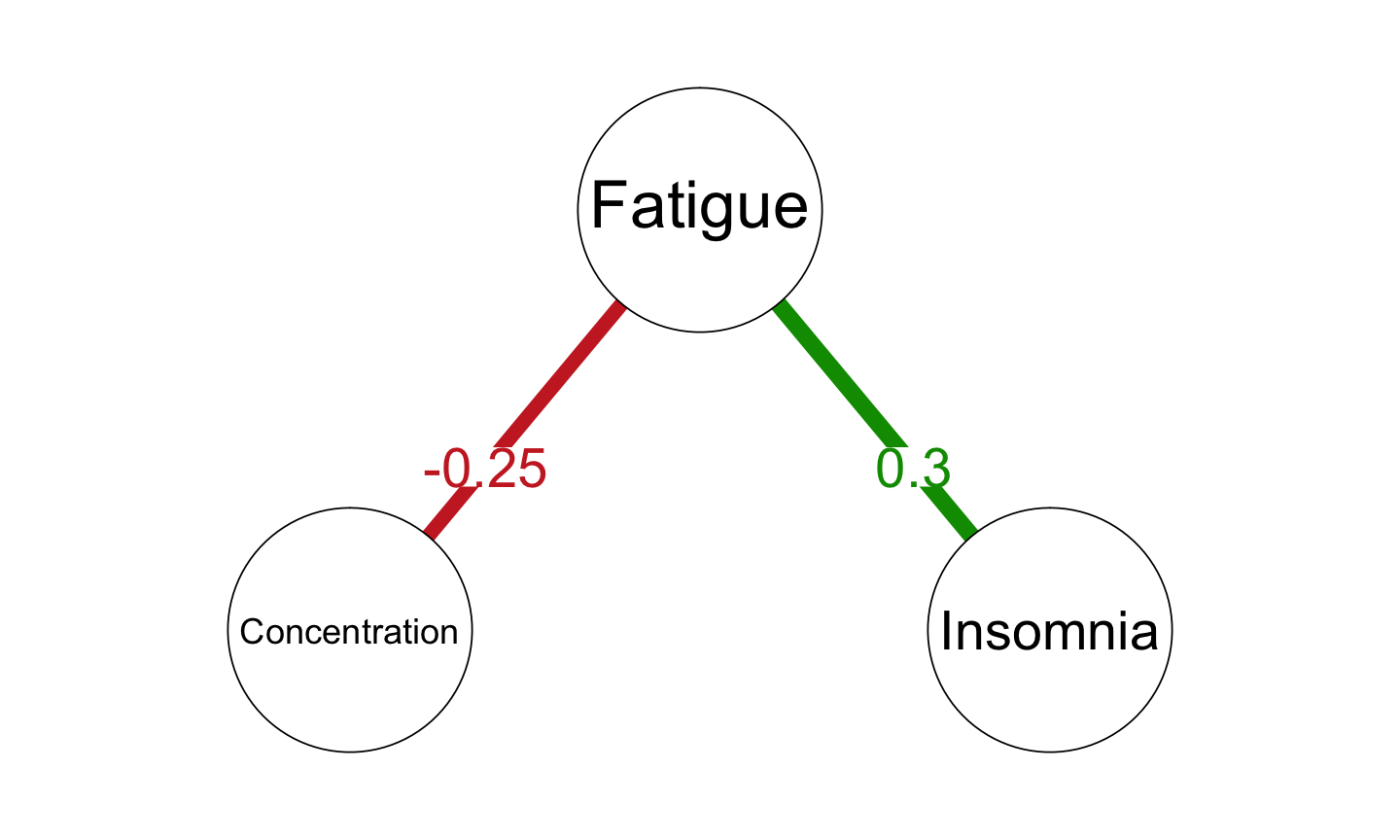• GGM is a network of partial correlation coefficients:
• $$\mathrm{Cor}\left(Y_i,Y_j \mid \pmb{Y}^{-(i,j)}\right) = - \frac{\kappa_{ij}}{\sqrt{\kappa_{ii}} \sqrt{\kappa_{jj}}}$$

The GGM model:

• Concentration $$-$$ Fatigue $$-$$ Insomnia

Is equivalent to three causal structures:

1. Concentration $$\rightarrow$$ Fatigue $$\rightarrow$$ Insomnia
2. Concentration $$\leftarrow$$ Fatigue $$\rightarrow$$ Insomnia
3. Concentration $$\leftarrow$$ Fatigue $$\leftarrow$$ Insomnia

Thus, the GGM highlights potential causal pathways

## GGM and Multiple Regressions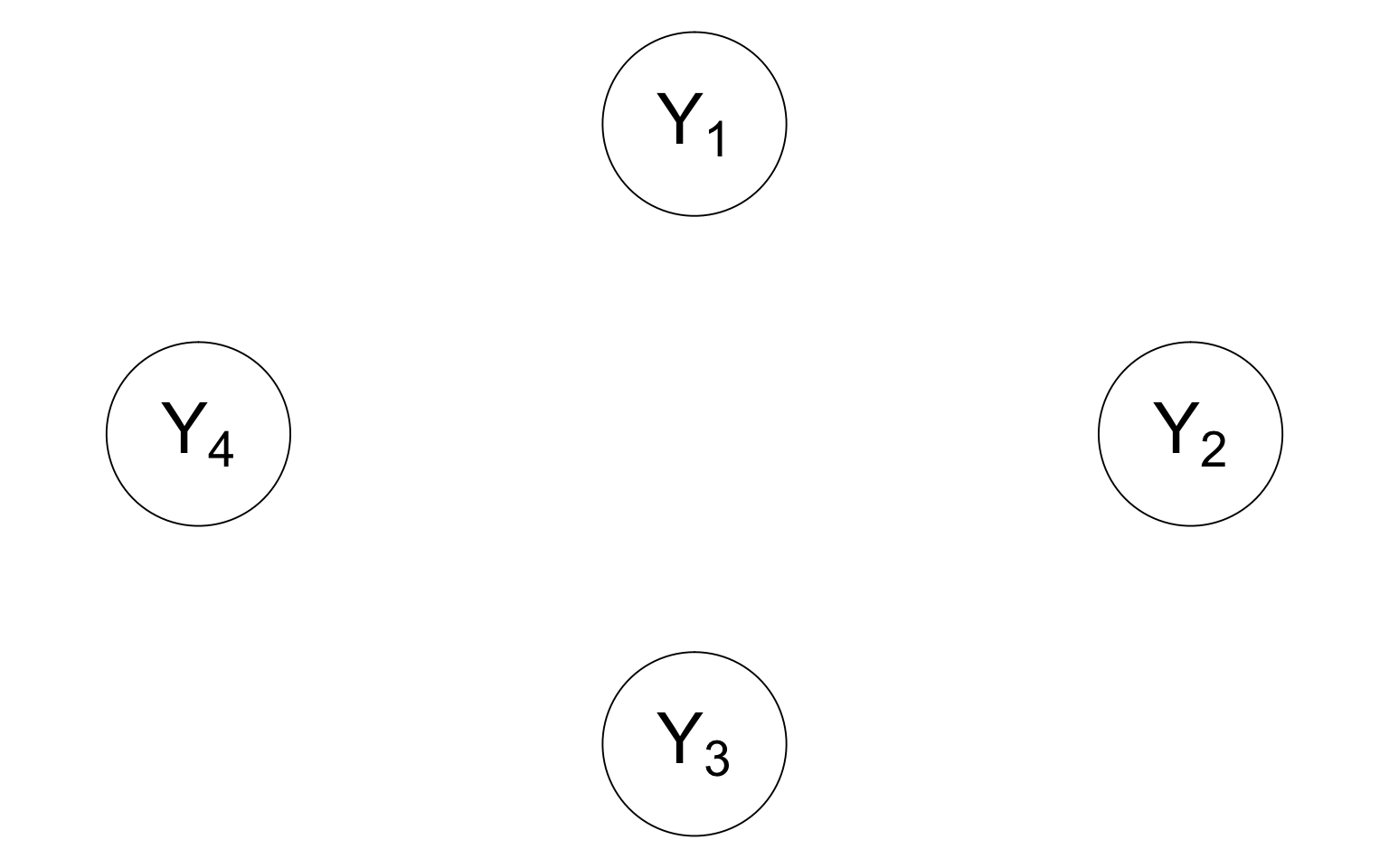## GGM and Multiple Regressions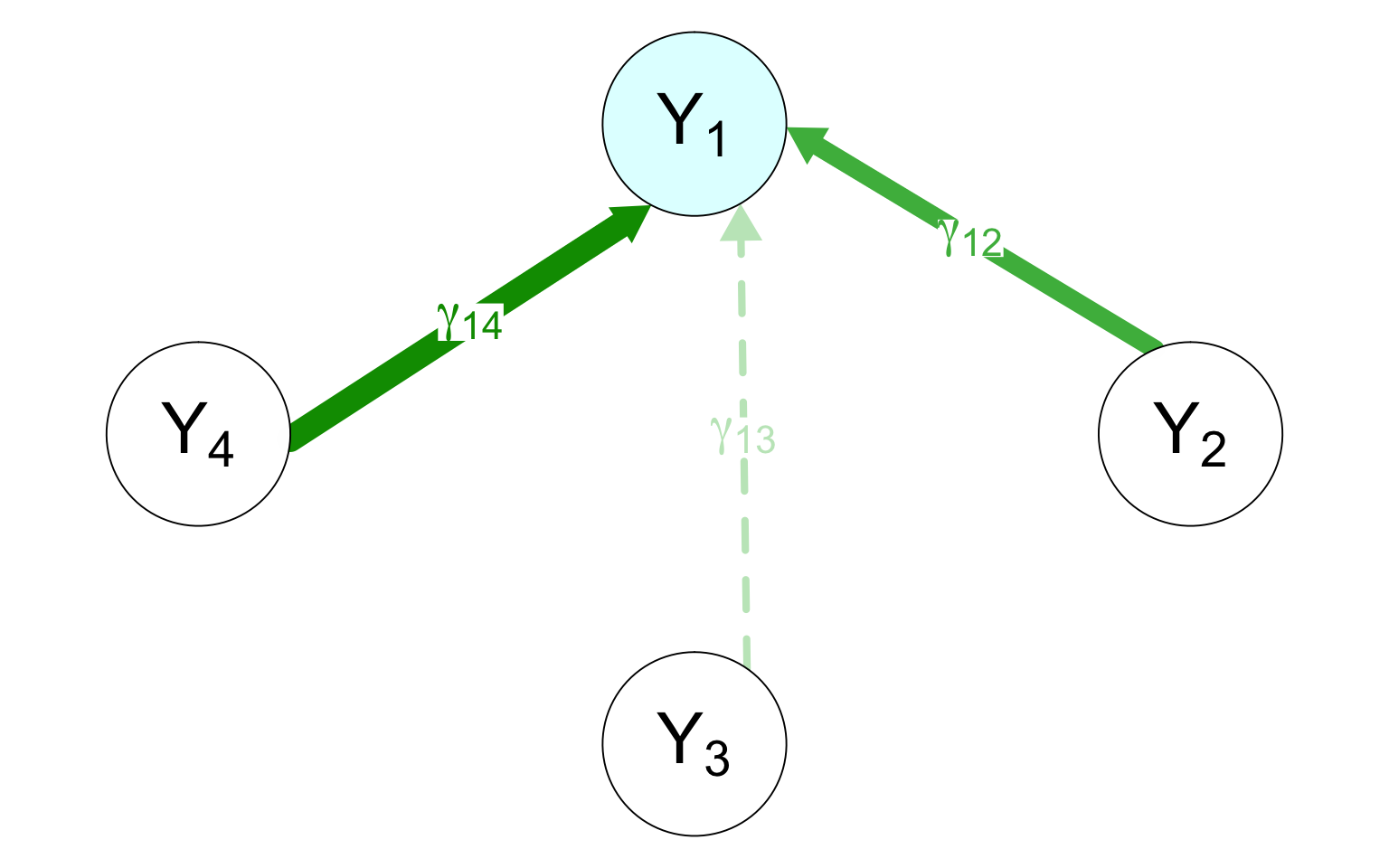$y_1 = \tau_1 + \gamma_{12} y_2 + \gamma_{13} y_3 + \gamma_{14} y_4 + \varepsilon_1$

## GGM and Multiple Regressions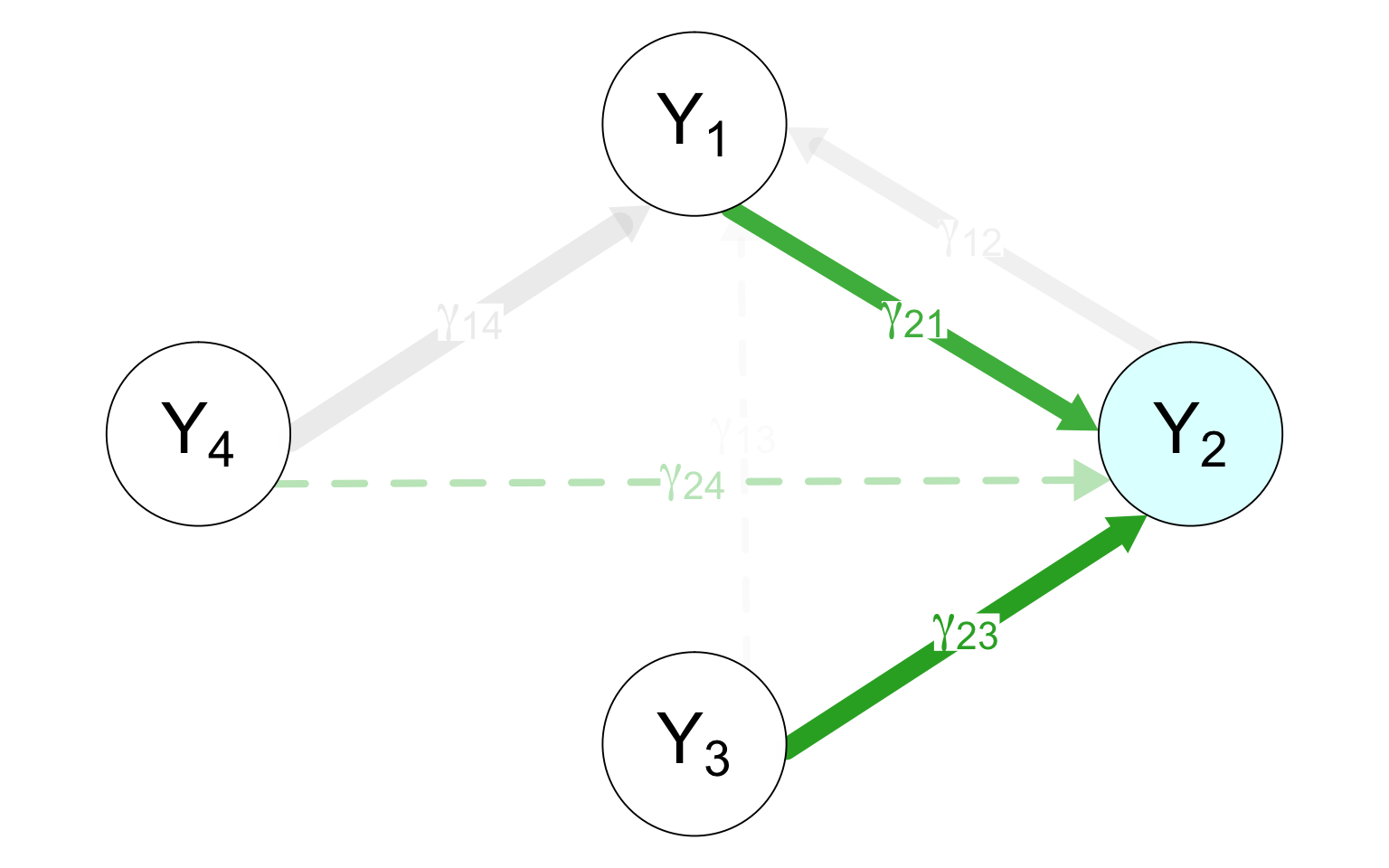$y_2 = \tau_2 + \gamma_{21} y_1 + \gamma_{23} y_3 + \gamma_{24} y_4 + \varepsilon_2$